Reversible Cellular Automata By Kwanghyun Paek

Home | Links | Applets | Papers | Class Plan | Team

 Research Paper

Click here for Presentation Slide

# Title: Reversible Cellular Automata

## 1.Introduction

Reversibility is a universal characteristic of physical law, and it is a precondition for the Second Law of thermodynamics to hold. Cellular Automata (CA) have certain basic features of the physical laws, such as uniformity and locality. While most CA are not reversible by themselves, we can program reversibility in CA so that we can observe the validity of the Second Law within CA. Wolfram illustrated many examples of CA that are reversible. Although it might seem that only CA with very simple behavior could be reversible, in fact, some CA with complex behavior can have reversibility. According to the Second Law, entropy-a measure of the disorder in a system-should increase in a reversible system. In other words, randomness of a system increases as the system evolves. However, Wolfram  discovered that rule 37R does not obey the rule. Rule 37R does not evolve to completely random equilibrium, nor does it show simple predictable behavior; it continues to fluctuate. Wolfram claims that this self-organization phenomenon explains how certain parts of the universe become progressively more organized despite of the Second Law.

## 2.Entropy and the Second Law

In the 1860s Rudolf Clausius explained entropy as a ratio of heat to temperature. More precisely, entropy was defined as heat absorbed by a system, divided by the absolute temperature of the system. Entropy of a system increases when it absorbs heat; respectively, entropy decreases when a system loses heat. As heat is associated with motion of atoms, an increase in entropy further implies increasing randomness of motion of atoms. Clausius and Thomson originally formulated the Second Law of thermodynamics in terms of that heat does not spontaneously flow from a colder body to a hotter one. It can again be interpreted as that entropy in a system tends to increase as heat spontaneously flows from hotter regions to colder regions in the system, and eventually the system evolves to completely random equilibrium. In order to run a system backward, all information related to evolution of the system should be maintained. In terms of thermodynamics, information is motion of atoms in a system, which is consequently entropy of the system. It will be impossible to restore the past of a system, if part of entropy of the system has turned into heat and flowed out of the system. By using cellular automata, a completely closed system can be simulated without loosing information. Legitimacy of tendency toward complete equilibrium in a reversible system therefore can be observed using cellular automata.

## 3.Reversible Cellular Automata

A cellular automaton is reversible (also called invertible or microscopically reversible), if its global map is invertible, i.e., for every possible state of the cellular automaton the global map specifies one and only one successor. If a cellular automaton is invertible, it therefore is deterministic in both directions of time. The rule that makes a cellular automaton go backward is called the inverse rule opposite to the direct rule that runs the cellular automaton forward direction of time. In general, the inverse rule is different from the direct rule except for trivial cases shown in the following figure.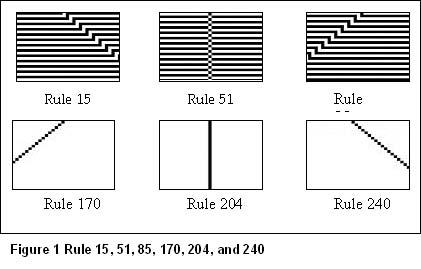In special cases, a cellular automaton can run backward under the direct rule by using the final state of the forward run as its initial state. This property is called time reversal invariant, and it is a stronger property than invertibility. Wolfram represented reversible cellular automata in which the new state of a cell is determined not only by the cell itself and its neighbors one step back but by the cell itself two steps back. Although those reversible cellular automata were named following the original cellular automata by Wolfram, the reversible cellular automata are not the invert rules of the original ones. In fact those reversible cellular automata are rather new kind ones having the two steps back cell as an additional neighbor that affects the current state of the cell. In the strict sense, those reversible cellular automata are time reversal invariant of which rule has built-in invertibility. Figure 2 shows some examples of the reversible cellular automata from Wolfram. They follow the original rules, but new state of a cell is inverted if the cell was black two steps back.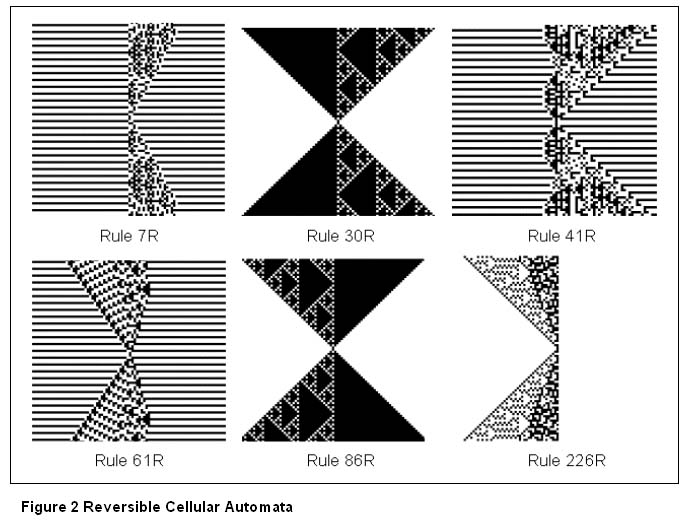The technique used here generating reversible cellular automata can be applied to all one-dimensional cellular automata; however, no general procedure is known to determine whether a certain rule has an inverse rule. Some techniques for constructing invertible cellular automata were presented by Toffoli and Margolus .

## 3.1.Second-order Technique

Second-order technique constructs time reversal invariant systems. Toffoli and Margolus gave a very easy explanation of the general idea of this method.

"If we cut a single frame out of the movie of a flying bullet, we have no way of knowing what the bullet is doing. However, if we are given two consecutive frames, then we can figure out the bullet's trajectory. That is, from there two frames, interpreted as the bullet's "past" and "present" positions, we can construct a third frame giving the bullet's "future" position; this procedure can be iterated." 

This method needs to keep the current and the previous states of each cell. The new state of each cell is determined as a linear combination of the current and the previous states. In the following formula, x is a given arbitrary function, q is the state, and t is the time step.

```
q^(t+1) = xq^t - q^(t-1)

```

Constructing the reverse step is straightforward by rearranging the above formula.

```
q^(t-1) = xq^t - q^(t+1)

```

Wolfram's time-reversal invariant rule exactly follows this method. Instead of subtraction, it simply does XOR the outcome of the original rule (xq^t above) and the previous configuration of the cell (q^(t+1) above). When cells have n states, mod n can be used instead. Following figure shows how Rule 30R differs from Rule 30.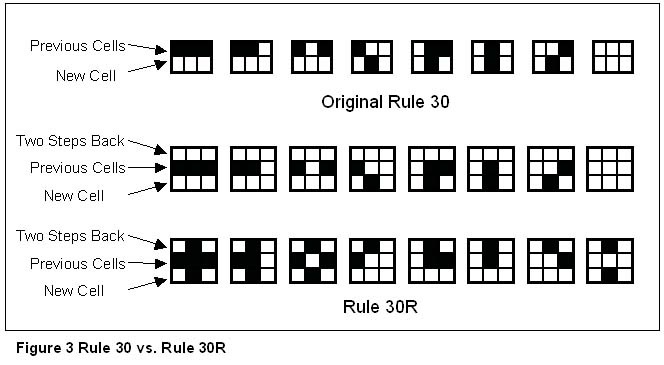Note that Rule 30R does exactly the same behavior when the cell was 0 or white two steps back, whereas state of new cell is flipped when the cell itself was 1 or black two steps back. To run a reversible cellular automaton backward, we only need to copy two steps back cells to the next step. Thereafter, the cellular automaton will rebuild the past of the system as shown in Figure 4.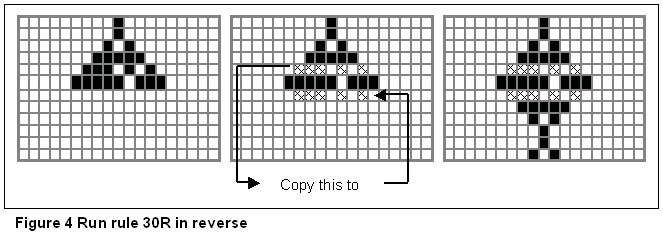## 3.2.Partitioning Technique

Partitioning is another technique to generate invertible cellular automata. Margolus neighborhood, named after Norman Margolus, is the well-known partitioning technique. The idea is that conventional cellular automata are in general not invertible because their reverse step is not deterministic. As we can see in Figure 3, four possible past configurations exit for either state of the current cell in conventional Rule 30. Obviously, it is impossible to guess the past step with the current state of each cell. The substantial reason is that conventional cellular automata may loose some information as evolving. For instance, shown in Figure 5, conventional one-dimensional cellular automata have three inputs, the cell itself and two neighbors, but the output is only one cell. Having the same number of states, it is very doubtful that the one output cell can preserve all the information of the three previous input cells. It is true that each cell affects three next-step cells, and the previous information could be distributed to the next-step cells conserving the total amount of information. However, the possibility of this happening does not seem very high. Here the Margolus neighborhood comes in shown in Figure 5. It uses as inputs the two cells of a block and returns as outputs the new states of all two cells of the same block; therefore, preserving information can be assured. If exactly the same partitioning scheme were to be used repeatedly, information would be unable to propagate crossing each individual partition. To prevent this, the Margolus neighborhood alternately groups odd and even cells every other step.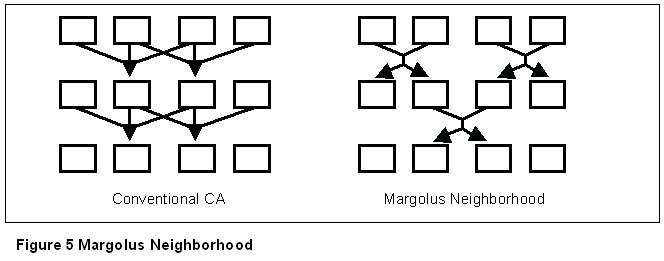The Margolus neighborhood has been expanded to two-dimension. Figure 6 show how the partitioning is done in two-dimension, and Figure 7 is an example of Billiard-Ball Model that follows the technique. Tim Tylor modified Marolus's two-dimension into a hexagonal model, called Q*Bert neighborhood.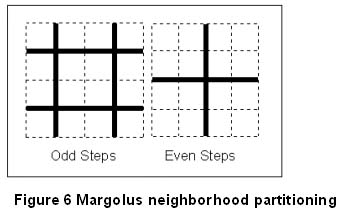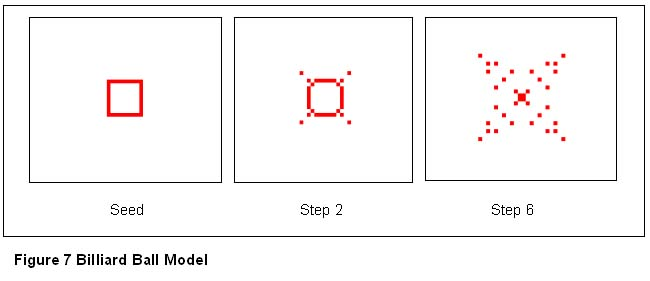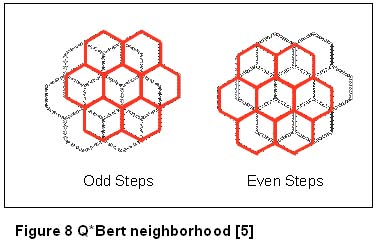## 4.Rule 37R

Wolfram introduced Rule 37R, which is a time- reversal invariant cellular automaton. While most reversible cellular automata he observed seemingly follow the Second Law of thermodynamics, he found out that the Rule 37R behaves in a different way. Instead of evolving to complete equilibrium, the Rule 37R manages the complexity of its structure and continues to fluctuate. In addition, it has localized structures that fluctuate and occasionally emit small sub-structures, called membranes. Those membranes are received by neighboring structures, which seems they are communicating each other.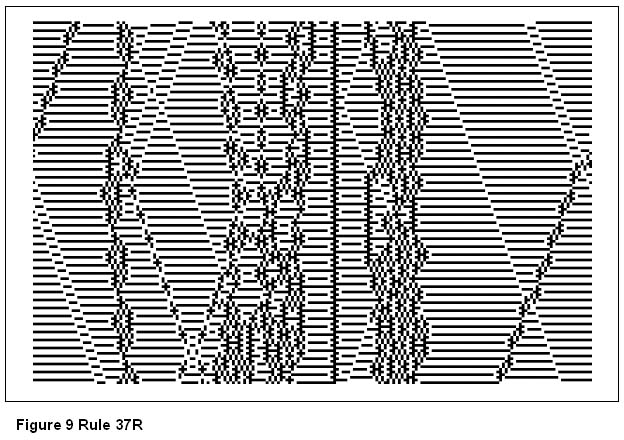By giving the facts mentioned earlier, Wolfram claimed that the Rule 37R does not obey the Second Law. It is true that the Rule 37R never settles down no matter how long we let it run. Wolfram said that the behavior of the Rule 37R is consistent with that of the universe. He pointed out that the universe has not evolved to completely random equilibrium even though it microscopically follows the Second Law. In fact, we have observed that certain parts of the universe are still becoming more organized arranging new galaxies and composing new planets. Wolfram also tried to explain the membranes of the Rule 37R in comparison with the radiation emitted and absorbed in the universe.

## 5.Discussion

Most fundamental physics and thermodynamics rules including the Second Law have been researched based on gas models. The reason is that gases not only compose majority of the universe but also obey the most fundamental rules we have discovered. In gas models, the First Law, energy is conserved, represents that the number of molecules remains in a gas model, and the Second Law, heat does not spontaneously flow from a colder body to a hotter one, implies that the motion of molecules progressively becomes more and more random. Many people have proved the universe does follow the two rules microscopically; however, we have not seen solid evidences whether the universe does so macroscopically. The question is that why the universe has not become complete equilibrium despite of the implication of the Second Law.

We have discussed earlier about the legitimacy of using cellular automata to observe the universal behavior; it is a closed system, has built-in uniformity and locality, and is able to have reversibility. As Wolfram claimed, the Rule 37R does not obey the Second Law. Nevertheless, it is hard to believe that the behavior of Rule 37R explains the universe. Not only does the Rule 37R disobey the Second Law, but it disobeys the First Law. The number of molecules in a gas model can be interpreted as the number of black cells in a cellular automaton. As we have seen in Figure 9, the number of black cells changes as the system evolves. It is doubtful that a model of which molecules' origin is not clear can simulate the universal behavior. Wolfram has noticed this issue, and tried to find a rule that obeys the First Law. He expanded his research to next-nearest-neighbor rules as he failed to find any non-trivial one from nearest-neighbor rules. Figure 10 shows what Wolfram found from next-nearest-neighbor rules. The number of black cells remains the same each step. However, we do not see the complexity we have seen from the Rule 37R. In fact, complexity of this rule starts only when the cells hit the boundaries. Wolfram admitted that the rule would show a trivial behavior unless it has boundaries.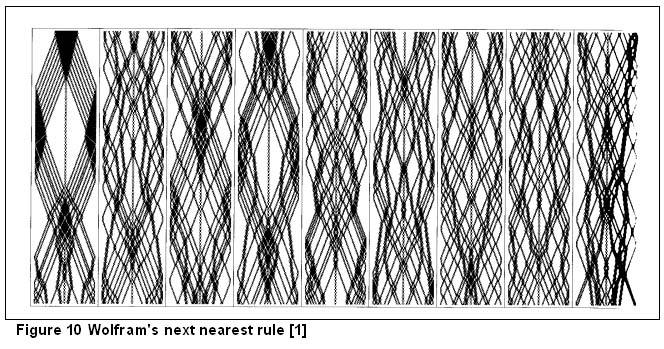## References

1. Wolfram, S. (2002). A New Kind of Science. Wolfram Media, Inc. IL. (pg. 435 ~ 457, 1017 ~ 1021).
2. Tommaso Toffoli and Norman Margolus. (1990). Invertible cellular automata: A review. Physica D 45. (pg 229-253).
3. Toffoli, Tommaso. (1980). Reversible Computing. Automata, Languages, and Programming (de Bakker and van Leeuwen ed.). Springer-Verlag. (pg. 632-644).
4. Toffoli, Tommaso and Margolus, Norman. (1987). Cellular automata machines: a new environment for modeling. MIT Press. Cambridge, Mass.

5. ## WWW Resources

6. Tylor, Tim. Cellular Automata. http://cell-auto.com/
7. McIntosh, Harold V. Reversible Cellular Automata. http://delta.cs.cinvestav.mx/~mcintosh/newweb/ra/ra.html

All NKS-SJSU Applets are Open Source Shareware, Papers are Copyrighted to their Authors.